Dettagli della rivista
Formato
Rivista
eISSN
1891-5469
Prima pubblicazione
01 Jan 2010
Frequenza di pubblicazione
1 volta all'anno
Lingue
Inglese
Accesso libero

# Magnetic induction pneumography: a planar coil system for continuous monitoring of lung function via contactless measurements

###### Ricevuto: 11 Oct 2010
Dettagli della rivista
Formato
Rivista
eISSN
1891-5469
Prima pubblicazione
01 Jan 2010
Frequenza di pubblicazione
1 volta all'anno
Lingue
Inglese
Introduction

Real time monitoring of lung function is of particular interest to the mechanically ventilated patients during critical care. In most cases, the patients remain immobilized in supine position for long periods of time. As a result of this recumbent position, a marked amount of fluid is accumulated in the posterior parts of the lungs due to gravity. Unless diagnosed early and treated successfully, the fluid accumulation leads to impaired gas exchange and may cause a respiratory failure. One common way to diagnose the fluid accumulation in the lungs is to have an x-ray examination of the patient, still, it requires the transport of the patient and does not allow for real time monitoring. Despite its low spatial resolution, electrical impedance tomography (EIT), a noninvasive and inexpensive device, is regarded as a promising approach to continuously monitor the ventilation distribution . The technique is based on the fact that, during ventilation, the lungs are periodically filled up with air which results in an increased impedance at the regions where the lung tissue is present. Therefore, local impedance variations indicate that the lung tissues at these regions are normally functioning. That way, EIT images has the potential to guide clinicians to optimize lung recruitment and maintain a reliable medical prognosis. However, notwithstanding the considerable improvements in the last decade, using electrodes in direct contact with the skin yet induces serious problems with regard to the poor skin-electrode contact  and the electrode position uncertainties . Besides, depth sensitivity gets worse if there exists a poorly conducting layer, i.e., bone tissue, on the surface . Additional side-effects of using electrodes may be irritated skin or even an allergic reaction, particularly for the infants and patients with sensitive skin.

Recent studies have shown that magnetic induction measurements with single coils provide signals which are correlated with the lung dynamics . However, for regional monitoring, in principle, it is essential to collect a set of measurements from different sites to be used in image reconstruction. Therefore, this idea is extended here by using coil arrays for data acquisition in order to image the regional thoracic conductivity changes. By doing so, the contact problems faced in EIT can readily be eliminated and the patient comfort can be improved. Ona major drawback of using coils is the image degradation caused by the uncertainties of the skin positioning with respect to the coils . The proposed sensor configuration which is comprised by 25 coils in a 5 by 5 planar arrangement is illustrated in figure 1. The sensor system is assummed to be mounted onto the patient bed and will be used to expose the body and record the measurements. With this configuration, the measurement cables can easily be installed through holes drilled in the bed.

Although, the physical nature of the problem is equivalent to that of magnetic induction tomography (MIT) [7, 8], which is another impedance imaging modality alike EIT, the sensor configuration differs significantly from MIT. Considering also that the principal focus is monitoring of the regional lung dynamics, the proposed technique will be referred to as magnetic induction pneumography (MIP). In MIP, the data are acquired by successively exciting each coil in order to induce an eddy-current density within the dorsal tissues and measuring the corresponding response magnetic field strength by the remaining coils. The recorded set of data is then used to reconstruct the internal conductivity distribution by means of algorithms that minimize the residual norm of the difference between the estimated and measured data . Regularization methods are typically applied to stabilize the image reconstruction process .

To simulate the measurements, an eddy-current finite element solver was used to compute the electromagnetic fields. The sensitivity maps were obtained by computing the adjoint fields according to Geselowitz’s reciprocity theorem . For the assessment of the technique, the sensitivity maps for each transmitter-receiver coil pair were studied. The spatial resolution of the configuration was investigated by examining the point spread functions (PSF) at different locations and depths. To simulate a realistic scenario, a chest model was generated by segmenting the tissue boundaries from NMR images. The electrical properties were obtained from the literature . Truncated singular value decomposition (SVD) was performed in order to reconstruct the images. The reconstructions of the ventilation distribution and the development of an edematous lung injury were presented. The imaging artifacts caused by either the incorrect positioning of the patient or the expansion of the chest wall due to breathing were studied by simulations.

Methods
Simulation of the voltages

The forward problem is defined as, given the electrical properties of the body and the geometry of the coil system, solving for the induced voltages in the receiver coils. This requires the solution of Maxwell’s electromagnetic equations which was reported in several earlier studies (see, for example ). A brief review is given here to provide a background and set the stage for the inverse problem.

Assume an AC driven coil located in the proximity of a volume conductor which is represented by Ω. The forward problem is to compute the induced voltages in the remaining pick-up coils due to the eddy-currents in Ω. According to Geselowitz’s reciprocity theorem, the induced voltage can be expressed as follows,

$v=−∫ΩσE1σ⋅E2σdV,$$$v=-\int_{\Omega }{\sigma {{\mathbf{E}}_{1}}\left( \sigma \right)}\cdot {{\mathbf{E}}_{2}}\left( \sigma \right)dV,$$

where E1(.) and E2(.) represent the direct and adjoint electric fields induced in the body, respectively. E1(.) is computed directly from the field of the driven coil and E2(.) is computed assuming a unity current in the pick-up coil as if it acts as a transmitter coil. σ represents the electrical conductivity distribution within Ω. The computation of E1(.) and E2(.) requires the solution of elliptic partial differential equations and the computational details can be found in an earlier study .

Differential measurements

In MIP and all related impedance imaging techniques, applying a differential measurement strategy is favorable because the systematic errors and drifts are better suppressed in this way. However, if the measurements are taken at different operating frequencies, unlike EIT, a scaling is necessary  as given below,

$Δva,b=va−wa/wb2vb.$$$\Delta {{v}_{a,b}}={{v}_{a}}-{{\left( {{{w}_{a}}}/{{{w}_{b}}}\; \right)}^{2}}{{v}_{b}}.$$

In here, va and vb, respectively, represent two sets of voltages for two distinct conductivity distributions, σa and σb, acquired at angular frequencies wa and wb. If the data are acquired at the same frequency, as in the case of time-differential measurements, then the scaling factor (wa/wb)2 becomes unity.

Sensitivity of measurement to conductivity

Presently linearization approaches are commonly used to deal with the nonlinearity of the forward map. By linearizing (1), the change of voltage data, ᐃv, due to a conductivity perturbation, ᐃσ, around a particular distribution, σ, can be approximated from ,

$Δv=−∫ΩΔσE1σ.E2σdV.$$$\Delta v=-\int_{\Omega }{\Delta \sigma {{\mathbf{E}}_{1}}\left( \sigma \right).}{{\mathbf{E}}_{2}}\left( \sigma \right)dV.$$

When a piecewise constant conductivity distribution, i.e., σ = [σ12, · · · ,σN]T , is assumed within Ω, (3) can be expressed as,

$Δv=−∑e=1NΔσe∫ΩeE1σ.E2σdve,$$$\Delta v=-\sum\limits_{e=1}^{N}{\left( \Delta {{\sigma }_{e}}\int_{{{\Omega }_{e}}}{{{\mathbf{E}}_{1}}\left( \sigma \right).{{\mathbf{E}}_{2}}\left( \sigma \right)d{{v}_{e}}} \right)},$$

where N is the number of voxels, i.e., the number of elements in the FE mesh. By introducing S(.) as the sensitivity matrix, (4) can be expressed by a linear matrix equation which relates the perturbation in the conductivity distribution to the perturbation in the voltage data,

$Δv=SΔσ.$$$\Delta v=\mathbf{S}\Delta \sigma .$$

The entries of S are obtained by successively computing the integral in (4) for different pairs of transmit-receive coil. For instance, assuming M transmitter and N pick-up coils, S consists of M × N rows, each of which corresponds to an independent set of voltage data. It should be noted that this approximation is valid only for limited conductivity fluctuations around σ for which the linear approximation error stays below a defined threshold.

Image reconstruction

The image reconstruction problem is to restore the conductivity perturbation, $Δσ^,$$$\Delta \hat{\sigma },$$from the measurement data, ᐃv. By applying SVD to the sensitivity matrix, i.e., S = UΣVT , and performing the inverse, $Δσ^$$$\Delta \hat{\sigma }$$can be approximated as follows,

$Δσ^=V∑t†UTΔv.$$$\Delta \hat{\sigma }=V\sum\nolimits_{t}^{\dagger }{{{\mathbf{U}}^{T}}\Delta v.}$$

In here, U and V are the singular vectors which form a basis for the data and the model space, respectively, and Σ is a diagonal matrix, the diagonal entries of which are the reciprocal of the singular values. Due to the ill-conditioning of S, which can be noted from the fast decay of singular values presented in figure 2, a truncation by using only the t column vectors of U and V corresponding to the t largest singular values is preferred to stabilize the inversion .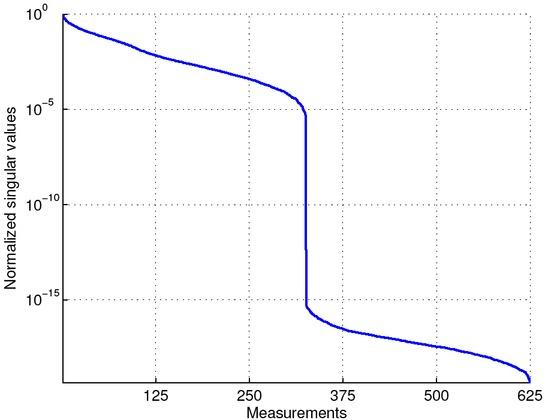The normalized spectrum of the sigular values. The exponential decay illustrates the ill-posedness of the image reconstruction.
Regularization

Let ρ1 be the largest singular value and ρt the tth largest one, the proper selection of the truncation level t, was performed by selecting the maximum t that satisfies the following equality:

$SNR=20log10ΔvRMSδRMS≥20log10ρ1ρt,$$$\text{SNR=20lo}{{\text{g}}_{10}}\left( \frac{\Delta {{v}_{RMS}}}{{{\delta }_{RMS}}} \right)\ge 20{{\log }_{10}}\left( \frac{{{\rho }_{1}}}{{{\rho }_{t}}} \right),$$

where ᐃvRMS and δRMS are the root-mean-square of the perturbed voltage signal and noise signal, respectively. The left-hand side is referred to as the SNR. When the noise is considered to be additive white Gaussian noise (AWGN) with zero mean, then, δRMS denotes the standard deviation of the noise signal.

Image resolution

Assuming that the measurement data are uncorrelated and the data variances are normalized to unity, the image resolution can be expressed in terms of the SVD components as follows ,

$R=VΣt†ΣtVT.$$$\mathbf{R}=\mathbf{V}\Sigma _{t}^{\dagger }{{\Sigma }_{t}}{{V}^{T}}.$$

Thus, the resolution matrix is simply VVT considering only the singular vectors corresponding to the t largest singular values. Ideally, R would be an identity matrix, however, a perfect resolution can never be attained in practice due to the nonzero measurement noise. The columns of R are commonly referred to as the PSFs, which describe the disturbance in the reconstructed image due to a conductivity perturbation in a single voxel.

Coil arrangement

A 5× 5 planar coil arrangement used in simulations is depicted in figure 1. The solenoid coils were approximated as infinitely thin circular wires. They are assumed to act both as transmitters and receivers and have a radius of 2.5 cm. The sensors cover a square region of 625 cm2 in total. For the simulations, the approximate distance from the coil center to the body surface was 1 cm. Figure 3 illustrates the coil geometry used in simulations with respect to the 3-D chest model.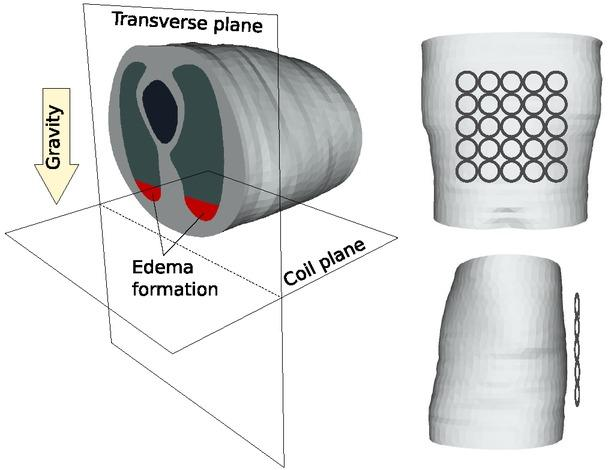The realistic chest model and the coil arranegement used in simulations. Red regions within the lungs represent the fluid accumulation, i.e., edema formation regions, due to gravity.
Chest model

The tissue boundaries of the chest model was segmented from the NMR images to construct a three dimensional volume. Typically, reconstruction of small details is infeasible with diffused imaging modalities; therefore, the model was constructed roughly by considering only the extended tissue groups such as the lungs, heart and the surrounding muscle tissue. Two different tetrahedral meshes with different sizes of approximately 100000 and 60000 elements were generated to be used independently for voltage simulation and image reconstruction, respectively. The electrical conductivities of the tissues were taken as 0.27, 0.11, 0.21 and 0.36 Sm−1 for deflated lung, inflated lung, heart and muscle tissues, respectively, corresponding to 100 kHz operating frequency .

The fluid accumulation that causes edematous lung injury takes usually place when the patients spend long time immobilized in supine position, and thus, gravitation forces a marked amount of fluid accumulate in the posterior parts of the lungs. The collapsed region is commonly a mixture of edematous fluid together with the deflated lung tissue causing the conductivity of that region to be higher than the healthy tissue. A conductivity value of 0.6 Sm−1 was assigned to the collapsed region according to . The formation of edema in the posterior regions of the lungs is illustrated as red regions in figure 3.

Results
Spatial sensitivity

The sensitivity matrix of the design was calculated assuming that the coil plane is located 1 cm above a half-space that has a uniform conductivity distribution. The sensitivity distributions for different coils in the sensor matrix are presented in figure 4. Both the sensitivity distribution near the surface and on the transverse plane are shown. For all setups, the region between the coils provides positive voltages, i.e., positive sensitive regions. The black lines represent the height and width of the regions where the sensitivity drops to 95% of the maximum sensitivity. The depth sensitivity increases with the increasing relative distance between the coils. However, further increase of the distance than 15 cm did not show any improvement in depth sensitivity.

The resolution matrix was computed using the same half-space model by assuming that the coil plane is located 1 cm above the half-space. To determine the truncation level necessary for a stable image reconstruction, the logarithmic ratio between the first and the remaining singular values as compared to the SNR levels was examined. The optimum truncation level for the designs corresponding to the SNR levels of 40 dB, 60 dB and 80 dB were found to be 145, 216 and 275, respectively. The PSFs of several locations for the SNR level of 60 dB are presented in figure 5. Near the surface regions, the PSFs are sharp and narrow, however, with increasing depth, they become wider and show more diffuse characteristics. Also the maximum amplitude of the PSFs approximately drops exponentially with depth. Besides, the peak of the PSFs shift towards the surface regions (more in deeper regions and less in surface-near regions) and thus, the localization errors become more prominent with increasing depth.

Ventilation monitoring

Assume that the patient is in a supine position and the measurements are taken at different times, t1 and t2. Using time-differential voltage measurements, i.e., ᐃv = v(t2) − v(t1), one can monitor the change of electrical conductivity in time due to breathing, i.e., ᐃσ = σ(t2) −σ(t1). The measurements were computed at t1 and t2, that correspond to the deflated and inflated lungs, respectively, while the thorax geometry is fixed. 3 sets of voltage data were generated by adding AWGN that correspond to 40 dB, 60 dB and 80 dB SNR levels. For the image reconstruction, the sensitivity matrix was computed based on the deflated lung state where the tissue boundaries can be segmented from high resolution imaging modalities. Thus, the drop in conductivity due to inflation of the lungs is aimed to be imaged. The optimal truncation parameter t was found to be 130, 227 and 300 for the corresponding SNR levels of 40 dB, 60 dB and 80 dB SNR, respectively. The reconstructed images due to the temporal conductivity changes during ventilation are presented in figure 7. The slices correspond to the transverse plane of the thorax model that is orthogonal to the sensor plane. Figure 6 illustrates the original model that corresponds to that slice. The recontructed images in figure 7 show blurry characteristics, however, both lung regions can be seperately identified at the dorsal region. Strong negative artifacts were noted between the lungs, particularly for the case of 60 dB and 80 dB SNR. Several positive and negative “spiky” artifacts were noted particularly near the dorsal surface.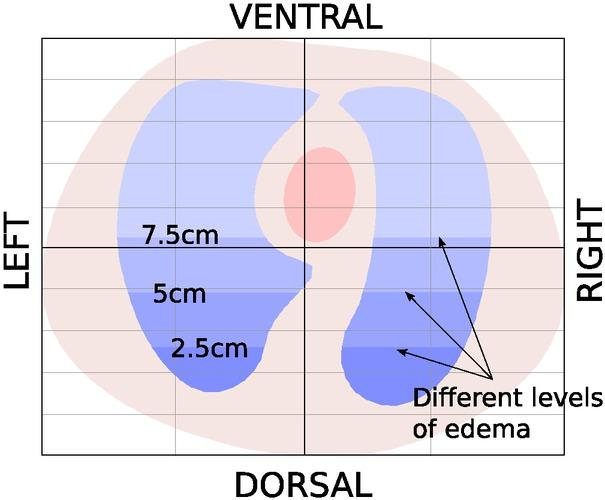The transverse plane (i.e., imaging plane) of the chest model. The color contrasts of blue in the lung regions represent the levels of edetamous lung injury at 2.5 cm, 5 cm and 7.5 cm.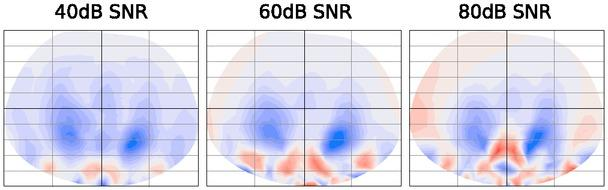The reconstructed images due to the temporal conductivity changes during ventilation for diffenrent SNR levels. The slices correspond to the imaging plane.
Assessment of heterogenous distributions

Is some cases, especially for the patients that spend long time in a supine posion, a markedly increased gravitational fluid accumulation hinders normal functioning of the lungs in the posterior regions. To simulate this, it was assumed that the fluid accumulation has reached a state in which 2.5 cm, 5 cm and 7.5 cm of the lower lungs have completely collapsed (refer to figure 6 for the illustration of levels). The differential measurements at t1 and t2 again correspond to the measurements for the case of deflated and inflated lungs, respectively, however, this time with the collapse. AWGN of 60 dB was added to the voltage data. Figure 8 shows the reconstructed images for different cases. The left column shows the lung function which has happened in both lungs simultaneously. The middle and right columns represent the cases that the accumulation develops faster in one lung than the other, i.e., corresponding to the collapse in the left lung and right lung only. The rows denote different levels of collapse. Since the conductivity of the collapsed region is time-invariant, the amplitudes of the collapsed regions get smaller. It is possible to visually inspect the difference in the distributions due to the considerable drop in amplitude.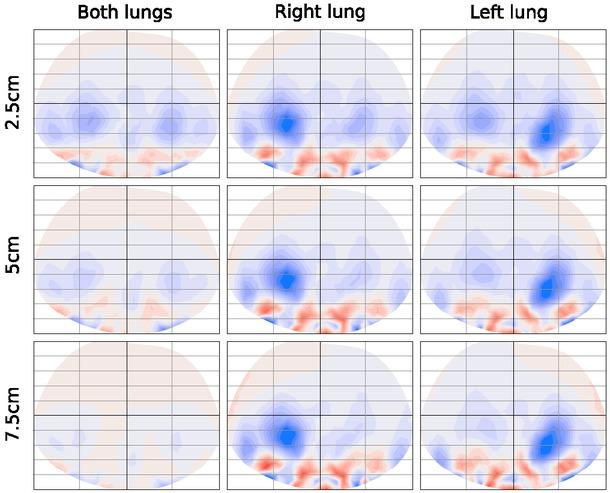The left column shows the lung function with different levels of lung collapse occured in both lungs simultaneously. The middle and right columns represent, respectively, the cases that the collapse occured in the left lung and right lung only. The rows denote different levels of lung injury.
Imaging of edematous lung injury

It is also possible to image the edematous lung injury by recording the initial measurements from the patient with healthy lungs at t1 and obtaining the differential measurements assuming that the fluid accumulation emerged at t2. Again, AWGN corresponding to 40, 60 and 80 dB SNR levels was added to the voltage data. Figure 9 shows the reconstructed edematous regions for different levels of collapse. The intensity of the reconstructed perturbation gets larger when increasing the volume of the edematous region. The “spiky” artifacts are again visible near the surface.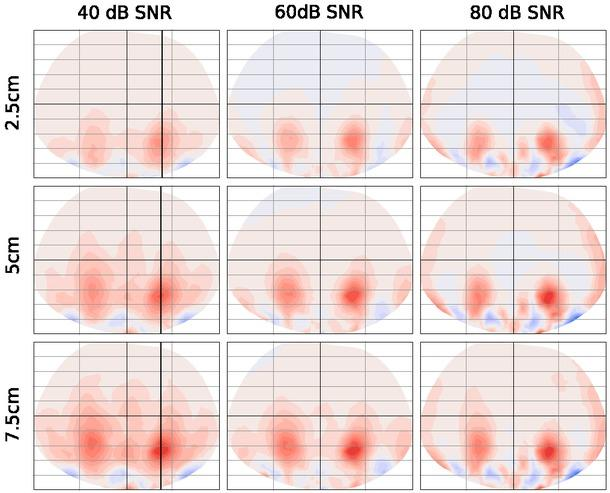Image reconstructions of the edematous region for different levels of collapse. The rows denote different levels of lung injury.

Figure 10, shows the normalized edema reconstructions along a line passing through the peak value of the reconstructed edema from ventral to dorsal, normal to the coil array plane, (see the red line in figure 9). The x-axis represents the ventral to dorsal orientation. The corresponding full-width-at-half-maximum (FWHM) values of the curves are illustrated as boxcar functions. It was found that the FWHMs provide marginal information on the level of the collapse, however, it is clearly visible that the amplitude drops drastically with the increasing volume of fluid accumulation. Thus, the variations in amplitude can be used to infer the development of fluid accumulation rather than the distributions.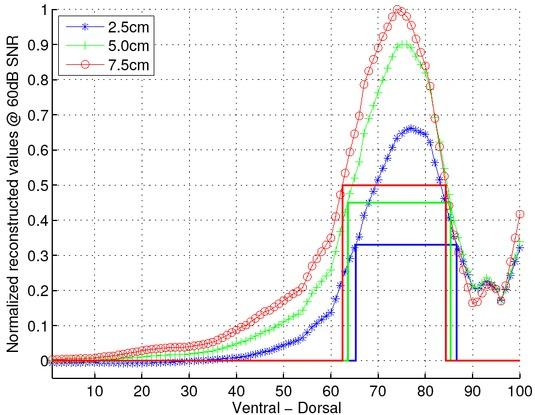The normalized edema reconstructions along the ventral to dorsal oriented line passing through the peak value of the reconstructions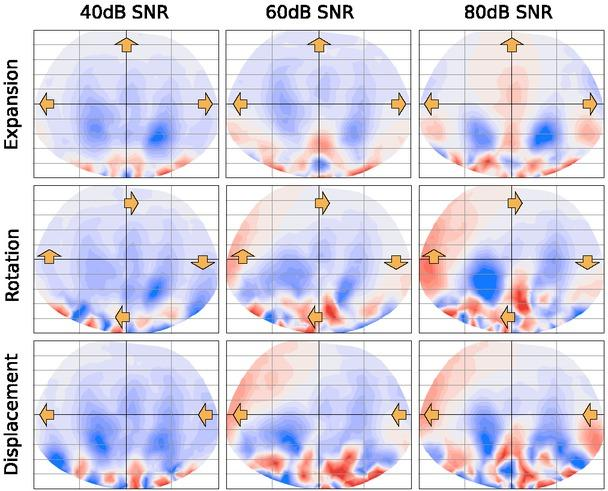Possible reconstructions that may be caused by different distorsions are illustrated. The rows denote, respectively, the images reconstructed from different body distorsions, i.e., 10% chest expansion, 10o clockwise rotation and 2 cm right-lateral displacement.
The effect of body distorsions on images

It is inevitable that the patients change the posture or position during ventilation which causes alterations in the measurement signal. It was previously reported that these alterations are noticed as serious artifacts in the images . To this end, we performed a simulation test to investigate the stability of image reconstructions to several body distorsions. The differential measurement were simulated by assuming that the body posture is modified at t2 as opposed to the state at t1. Figure 9 illustrates the possible artifacts that may be observed for different distorsions. The columns represent the images reconstructed from the voltage data with different SNR levels. The rows denote the images reconstructed from different body distorsions, i.e., 10% chest expansion, 10o clockwise rotation and 2 cm right-lateral displacement. The expansion of chest does not cause too severe artifacts and the lungs can still be reconstructed seperately. However, the rotation and displacement provide very poor reconstructions with many artifacts.

Discussions

The linearity assumption for the solution of the inverse problem may lead to undesired conductivity reconstructions when the change of the conductivity distribution is too large. This seems an important issue, particularly for lung applications, where the lung tissue is distributed extensively and the perturbations hardly fulfill the linearity assumption. Applying more iterations (i.e., Gauss-Newton iterations) may not always fix the problem, since the iterative algorithm usually converges to a local solution which may not be the desired one. A good strategy to overcome this problem is to select better conductivity distributions to be used for the computation of the sensitivity matrix. So far, uniform distributions have been used to attain the sensitivities, however by doing so, the imaging quality was found to be insufficient to clearly distinguish the right lung from the left lung . In this study, the sensitivity matrix was calculated by using an inhomogenous model, the tissue boundaries of which were segmented from NMR scans of the patient. Since many patients undergoing monitoring usually have these scans beforehand, this approach can easily be adopted. Such prior knowledge, as emphasized in this paper, increases the image quality, though, still severe artifacts can be noted especially near the boundary of the images.

The image reconstruction problem is severly ill-posed as can be noticed from the rapid decay rate of the singular values. Hence, regularization methods must be employed to overcome the ill-posedness and stabilize the image reconstruction. In this work, the truncation of the singular values was used for this purpose, however, there are several other approaches which have the potential to regularize the problem more efficiently. For instance, since the data acquisition is rapid and the successive data frames are strongly correlated, an appropriate strategy would be implementing a “Kalman filter” to take advantage of the temporal correlation in data frames within a reasonable time span . On the other hand, another way to improve regularization would be to use additional information based on the variability of the physiological parameters. It is known that the conductivity of the tissues varies with gender, age and condition of the patients, which renders it essential to determine the corresponding statistics to the model and to include this information as a prior assumption to regularize the inversion appropriately. This, in its simplest form, can be incorporated into the standard, Bayesian framework as a weighting term, i.e., a model covariance matrix, in the solutions.

It was previously reported for a complete magnetic induction tomography system that the artifacts due to breathing usually spoil the images . However, for the patient in a supine position, the chest motion due to respiration is assumed to take place only on the ventral surface and the dorsal movement artifacts are expected to be minimal for this position. This argument has been explored here and the artifacts due to breathing is found to be not as severe as in the complete tomography system. However, rotational and transversal displacements of the patient during data acquisition still cause significant artifacts. Nevertheless, considering the patients that are anestesized and stay immobilized in the ICUs, these rotational and displacement artifacts are not primarily influential. Thus, the images obtained with this planar system appears to be less sensitive to the motions than the complete tomography system.

In this work, the measurements were assumed to be insensitive to the cardiac cycles. The heart is placed in the ventral portion of the chest and, knowing that the sensitivity decays rapidly with depth, it is reasonable to neglect the corresponding effects. This can also be verified by analyzing the PSFs of the system. A conductivity perturbation at the location of heart shows a very distributed and smooth PSF with a very low amplitude and does not have a significant effect on the reconstructions of the dorsal regions. Nevertheless, it may be useful to apply a band-pass filter to supress signals correlated with the heart rate.

Conclusions

The feasibility of lung imaging using a planar coil matrix was explored. A realistic thorax model was constructed using NMR images and used for the analysis. This preliminary work points out the potential of the method to be used for real time monitoring of ventilation and fluidic changes. The sensor system can easily be mounted onto the patient bed. With this planar system, the sensitivity of the measurements drops rapidly with depth below the skin, therefore, the inhomogeneities in deeper regions can hardly be monitored. However, unless supressed via a filtering strategy, severe imaging artifacts can be induced due to the patient’s movement during data acquisition. For immobilized patients, the artifacts due to breathing is found to be not as severe as the complete tomography system. The future work may include finding optimal coil matrices so that the spatial resolution of the images is increased for particular locations which may provide better diagnostic information.

Muders T, Luepschen H, Putensen C. Curr Opin in Crit Care. 2010;16(3):269–275.10.1097/MCC.0b013e3283390cbfMudersTLuepschenHPutensenCCurr Opin in Crit Care201016326927520445445

Boone KG, Holder DS. Med Biol Eng Comput. 1996;34(5):351–354.10.1007/BF025200038945858BooneKGHolderDSMed Biol Eng Comput19963453513548945858

Gençer NG, Ider YZ. Physiol Meas. 1994;15(2A):A51–A57.808705010.1088/0967-3334/15/2A/007GençerNGIderYZPhysiol Meas1994152AA51A57Search in Google Scholar

Steffen M, Heimann K, Bernstein N, Leonhardt S. Physiol Meas. 2008;29(6):291–306.10.1088/0967-3334/29/6/S25SteffenMHeimannKBernsteinNLeonhardtSPhysiol Meas200829629130618544830

Gürsoy D, Scharfetter H. Physiol Meas. 2009;30(6):165–174.10.1088/0967-3334/30/6/S11GürsoyDScharfetterHPhysiol Meas200930616517419491439

Griffiths H. Meas Sci Technol. 2001;12(8):1126–1131.Griffiths H. Meas Sci Technol20011281126113110.1088/0957-0233/12/8/319Search in Google Scholar

Korjenevsky AV, Cherepenin VA, Sapetsky S. Physiol Meas. 2000;21(1):89–94.10.1088/0967-3334/21/1/31110720003KorjenevskyAVCherepeninVASapetskySPhysiol Meas2000211899410720003

Merwa R, Hollaus K, Brunner P, Scharfetter H. Physiol Meas. 2005;26(2):241–250.10.1088/0967-3334/26/2/023MerwaRHollausKBrunnerPScharfetterHPhysiol Meas200526224125015798237

Soleimani M. Int J Numer Anal Mod. 2008;5(3):407–440.SoleimaniMInt J Numer Anal Mod200853407440Search in Google Scholar

Mortarelli JR. IEEE T Bio-med Eng. 1980;BME-27(11):665– 667.MortarelliJRIEEE T Bio-med Eng1980BME-2711665–66710.1109/TBME.1980.3266777203506Search in Google Scholar

Gabriel S, Lau RW, Gabriel C. Phys Med Biol. 1996;41(11):2271–2293.893802610.1088/0031-9155/41/11/003GabrielSLauRWGabrielCPhys Med Biol19964111227122938938026Search in Google Scholar

Soleimani M. Int J Numer Anal Mod. 2008;5(3):407–440.SoleimaniMInt J Numer Anal Mod200853407440Search in Google Scholar

Gençer NG, Tek MN. Phys Med Biol. 1999;44(4):927–940.10.1088/0031-9155/44/4/00910232806GençerNGTekMNPhys Med Biol199944492794010232806

Brunner P, Merwa R, Missner A, Rosell J, Hollaus K, Scharfetter H. Physiol Meas. 2006;27(5):237–248.10.1088/0967-3334/27/5/S20BrunnerPMerwaRMissnerARosellJHollausKScharfetterHPhysiol Meas200627523724816636414

Hollaus K, Magele C, Merwa R, Scharfetter H. Physiol Meas. 2004;25(1):159–168.1500531310.1088/0967-3334/25/1/023HollausKMageleCMerwaRScharfetterHPhysiol Meas200425115916815005313Search in Google Scholar

Gürsoy D, Scharfetter H. Meas Sci Technol. 2009;20(10):105505 (9pp).GürsoyDScharfetterHMeas Sci Technol20092010105505910.1088/0957-0233/20/10/105505Search in Google Scholar

Tarantola A. Inverse problem theory and model parameter estimation. SIAM; 2005.TarantolaAInverse problem theory and model parameter estimationSIAM200510.1137/1.9780898717921Search in Google Scholar

Luepschen H, van Riesen D, Beckmann L, Hameyer K, Leonhardt S. IEEE T Magn. 2008;44(6):1450–1453.10.1109/TMAG.2007.916131LuepschenHvan RiesenDBeckmannLHameyerKLeonhardtSIEEE T Magn200844614501453

Gürsoy D, Scharfetter H. Journal of Physics: Conference Series. 2010;224(1):012039.GürsoyDScharfetterHJournal of Physics: Conference Series2010224101203910.1088/1742-6596/224/1/012039Search in Google Scholar